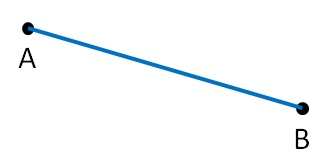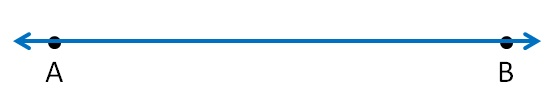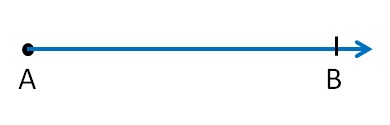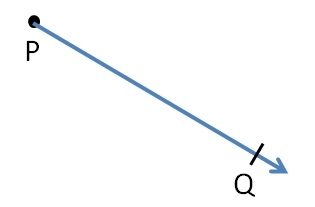If we join two points, we get line segment.We write line segment AB as (AB)  or (BA)

Here, points A and B are the end points of the line segment.

#### Line

A line extends in definitely in both directions.A line as no end point.

We write line  AB  as (AB)

#### Ray

Ray is a line with one end-point.Here AB is a ray.

It can extend from point B, but point A is fixed.

We write it as (AB)

Similarly,

(PQ) is a ray1. Chapter 5 Class 6 Understanding Elementary Shapes
2. Concept wise
3. Measuring Line Segments

Measuring Line Segments